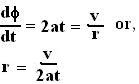## Tuesday, July 24, 2007

### Irodov Problem 1.44

If the radius of the wheel is given by r then we have,The tangential acceleration of the particle is given by,The normal (radial) acceleration is simply the centripetal acceleration and is given by,The total acceleration is thus given by,